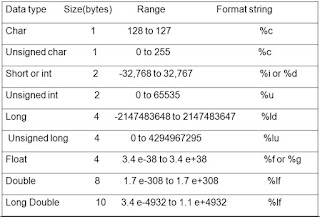## Wednesday, November 2, 2016

### INTEGER OVERFLOW - Altering the result of an arithmetic operation

0 - INTRODUCTION

- Integer Overflow happens when an arithmetic operation attempts to create a numeric value that is too large to be represented in its allocated storage space.

- In programming, a variable is a memory space reserved to store a value corresponding to a data type supported by the language. Programming languages ​​have several types of variables, and measurement memory space reserved for the variable depends on the type of variable that is defined.

- For example, ANSI C assigns these values to the Integer type:- If higher than permitted values ​​are assigned to char, short or int type variables, the program execution will not give any error, but truncate values.

- ISO C99 considers that the result of an integer overflow is of "undefined behavior", which means that standard compilers can do whatever they want, from completely ignoring the overflow to abort the program. What do most compilers is to ignore the integer overflow.

- The integer overflows can not be detected until they have occurred. This can be dangerous if the calculation has to do with the size of a buffer or the index of an array.

- Most integer overflow are not exploitable because memory is not being directly overwritten, but sometimes they can lead to other kinds of bugs. Integer overflow attacks will not allow to overwrite memory areas, variables or code, but they can change the application logic and even outflank memory structures created through unsafe variables. In other words, the result can be unexpected given the resulting value will not be provided according to the logic defined in the program.

- To prevent an integer overflow, checking numerical values must be comprehensive so that there are no unexpected errors, including a check to detect whether the entered values ​​are between a range of certain values, ​​and of course, check measurement data type before using it.

1 - INTEGER OVERFLOW EXAMPLE - Altering the result of an arithmetic operation

- Let's consider this program written in language C:

- The program accepts 3 arguments (int, int, char), sums the 3 of them and finally outputs the result.

-In case of proper input, arguments must be inside the range of accepted int and char type values. Let's see a normal operation for this program with values 1, 2, and 3:

- However, if the program is provided with arguments ​​which sum is outside the valid range, the sum exceeds the capacity of the outcome variable obtaining a negative number. For instance, let's see what happens with this input:

- How is it possible that the sum of those numbers is 0, instead of 2147483647 + 2147483647 + 2 = 4294967296

- Let's examine why the compiler considers that this sum is 0, instead of the expected result 4294967296.

- First of all it is important to notice that the number 4294967294 is out of the scope of the int type value range (-2147483648 to +2147483647).

- To understand the compiler's mind, we need to take the numbers written in a Two's Complement format, what is the usual way that negative numbers (starting by 1) are represented by compilers:

- For instance, in Two's Complement format the number 1101 would not be 13 d, but -3 d:

(-1)*2^3 + 1*2^2 + 0*2^1 + 1*2^1 = -3 d

- So, the int arguments (2147483647) of this example must be converted from decimal to Two's Complement binary system format:

- Then,  summing both up in a binary way:

0111 1111 1111 1111 1111 1111 1111 1111 = 2147483647 d
+
0111 1111 1111 1111 1111 1111 1111 1111 = 2147483647 d
------------------------------------------------------------------------------
1111 1111 1111 1111 1111 1111 1111 1110 = ?

- In the reverse way than previously, calculating the result of the binary sum 1111 1111 1111 1111 1111 1111 1111 11110 from Two's Complement format to decimal:

- So, the compiler takes the result of the partial sum as the negative -2. Then, the total sum would be 0:

(-2) +2 = 0

- Another possible integer overflow case ​​for the same program would be as follows:

- In this late case:

- Summing:

0111 1111 1111 1111 1111 1111 1111 1111 = 2147483647 d
+
0111 1111 1111 1111 1111 1111 1000 0010 = 2147483522 d
------------------------------------------------------------------------------
1111  1111 1111 1111 1111 1111 1000 0001= ?

- Converting the result from Two's Complement to decimal:

- The final total sum:

(-127) + 127 = 0